ASE Tweeter Full range Mids Woofer Subwoofer Car-HiFi no longer available
 Illuminator Revelator Classic Discovery

# ScanSpeak

Founded in 1970, Scan-Speak still resides at its original address in Videbaek, Denmark. Located in the heart of the Jutland peninsula, the acoustic engineers adhere to the legendary policy of "Never compromise".

## Woofer## Ellipticoropen a bigger photo

recommended cabinet 1:
closed cabinet with 1,9 L volume
from 134/84 Hz (-3dB/-8dB)

recommended cabinet 2:
5,1 L volume bass reflex cabinet
with HP50 reflex tube, 20 cm long.
from 76/58 Hz (-3dB/-8dB).

## ScanSpeak 18WE/4542T00

order no. ss-18WE4542T00

EUR 870,00excl. VAT: € 731.09 / \$ 811.51

17 cm bass-midrange driver with high quality cone.

• power handling (continuous/programme) = 70/130 W
• frequency range = 40-5000 Hz
• resonance frequency fs = 47 Hz
• impedance R = 4 Ohm
• sound pressure level SPL = 92,5 dB (2,83V; 1m)
• DC resistance Re = 3,4 Ohm
• force factor BL = 8,1 N/A
• voice coil inductance L = 0,1 mH
• effective piston radiating area Sd = 133 cm2
• effective mechanical mass incl. air load mms = 18,3 g
• equivalent volume of compliance Vas = 15,7 l
• total Q factor Qts = 0,27 (Qms=6,76, Qes=0,28)
• voice coil diameter = 35x45 mm
• maximum peak linear excursion vibration xlin = +/- 7,2 mm
• mounting diameter d = 158 mm
• overall diameter d = 180 mm
• mounting depth (not countersunk) t = 97,7 mm
PDF data sheet

## Illuminatoropen a bigger photo

recommended cabinet 1:
closed cabinet with 1,7 L volume
from 112/71 Hz (-3dB/-8dB)

recommended cabinet 2:
4 L volume bass reflex cabinet
with HP35 reflex tube, 18 cm long.
from 68/51 Hz (-3dB/-8dB).

## ScanSpeak 15WU/4741T00

order no. ss-15WU4741T

EUR 328,72excl. VAT: € 276.24 / \$ 306.62

14 cm bass-midrange driver with high quality cone. The magnet system is based on Neodymium technology.

• power handling (continuous/programme) = 80/150 W
• frequency range = 30-3500 Hz
• resonance frequency fs = 34 Hz
• impedance R = 4 Ohm
• sound pressure level SPL = 85,9 dB (2,83V; 1m)
• DC resistance Re = 3,2 Ohm
• force factor BL = 6 N/A
• voice coil inductance L = 0,5 mH
• effective piston radiating area Sd = 92 cm2
• effective mechanical mass incl. air load mms = 13 g
• equivalent volume of compliance Vas = 20,1 l
• total Q factor Qts = 0,23 (Qms=3,47, Qes=0,25)
• voice coil diameter = 42 mm
• maximum peak linear excursion vibration xlin = +/- 9 mm
• mounting diameter d = 123 mm
• overall diameter d = 149 mm
• mounting depth (not countersunk) t = 97,5 mm
PDF data sheetopen a bigger photo

recommended cabinet 1:
closed cabinet with 2 L volume
from 107/67 Hz (-3dB/-8dB)

recommended cabinet 2:
5,1 L volume bass reflex cabinet
with HP35 reflex tube, 15 cm long.
from 63/47 Hz (-3dB/-8dB).

## ScanSpeak 15WU/8741T00

order no. ss-15WU8741T

EUR 328,72excl. VAT: € 276.24 / \$ 306.62

14 cm bass-midrange driver with high quality cone. The magnet system is based on Neodymium technology.

• power handling (continuous/programme) = 80/150 W
• frequency range = 30-3500 Hz
• resonance frequency fs = 35 Hz
• impedance R = 8 Ohm
• sound pressure level SPL = 83,4 dB (2,83V; 1m)
• DC resistance Re = 5,9 Ohm
• force factor BL = 7,5 N/A
• voice coil inductance L = 0,41 mH
• effective piston radiating area Sd = 92 cm2
• effective mechanical mass incl. air load mms = 12,3 g
• equivalent volume of compliance Vas = 19,9 l
• total Q factor Qts = 0,26 (Qms=3,37, Qes=0,28)
• voice coil diameter = 42 mm
• maximum peak linear excursion vibration xlin = +/- 9 mm
• mounting diameter d = 123 mm
• overall diameter d = 149 mm
• mounting depth (not countersunk) t = 97,5 mm
PDF data sheetopen a bigger photo

recommended cabinet 1:
closed cabinet with 6,8 L volume
from 80/50 Hz (-3dB/-8dB)

recommended cabinet 2:
20 L volume bass reflex cabinet
with HP70 reflex tube, 27 cm long.
from 44/34 Hz (-3dB/-8dB).

## ScanSpeak 18WU/4741T00

order no. ss-18WU4741T

EUR 324,12excl. VAT: € 272.37 / \$ 302.33

17 cm bass-midrange driver with high quality cone. The magnet system is based on Neodymium technology.

• power handling (continuous/programme) = 80/150 W
• frequency range = 28-3500 Hz
• resonance frequency fs = 30 Hz
• impedance R = 4 Ohm
• sound pressure level SPL = 87,2 dB (2,83V; 1m)
• DC resistance Re = 3,2 Ohm
• force factor BL = 6 N/A
• voice coil inductance L = 0,5 mH
• effective piston radiating area Sd = 154 cm2
• effective mechanical mass incl. air load mms = 18,9 g
• equivalent volume of compliance Vas = 49,4 l
• total Q factor Qts = 0,29 (Qms=3,56, Qes=0,32)
• voice coil diameter = 42 mm
• maximum peak linear excursion vibration xlin = +/- 9 mm
• mounting diameter d = 158 mm
• overall diameter d = 184 mm
• mounting depth (not countersunk) t = 105 mm
PDF data sheetopen a bigger photo

recommended cabinet 1:
closed cabinet with 8,9 L volume
from 73/46 Hz (-3dB/-8dB)

recommended cabinet 2:
29 L volume bass reflex cabinet
with HP70 reflex tube, 21 cm long.
from 38/30 Hz (-3dB/-8dB).

## ScanSpeak 18WU/8741T00

order no. ss-18WU8741T

EUR 324,12excl. VAT: € 272.37 / \$ 302.33

17 cm bass-midrange driver with high quality cone. The magnet system is based on Neodymium technology.

• power handling (continuous/programme) = 80/150 W
• frequency range = 28-3500 Hz
• resonance frequency fs = 31 Hz
• impedance R = 8 Ohm
• sound pressure level SPL = 85,4 dB (2,83V; 1m)
• DC resistance Re = 5,9 Ohm
• force factor BL = 7,5 N/A
• voice coil inductance L = 0,41 mH
• effective piston radiating area Sd = 154 cm2
• effective mechanical mass incl. air load mms = 18 g
• equivalent volume of compliance Vas = 48,6 l
• total Q factor Qts = 0,33 (Qms=3,51, Qes=0,37)
• voice coil diameter = 42 mm
• maximum peak linear excursion vibration xlin = +/- 9 mm
• mounting diameter d = 158 mm
• overall diameter d = 184 mm
• mounting depth (not countersunk) t = 105 mm
PDF data sheet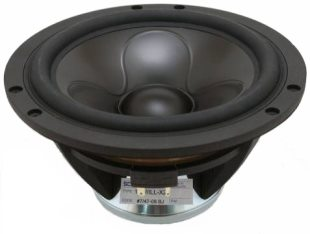open a bigger photo

recommended cabinet 1:
closed cabinet with 5,7 L volume
from 91/57 Hz (-3dB/-8dB)

recommended cabinet 2:
16 L volume bass reflex cabinet
with HP50 reflex tube, 13 cm long.
from 52/39 Hz (-3dB/-8dB).

## ScanSpeak 18WU/8747T00

order no. ss-18WU8747T

EUR 300,86excl. VAT: € 252.82 / \$ 280.63

17 cm bass-midrange driver with aluminium cone. The magnet system is based on Neodymium technology.

• power handling (continuous/programme) = 80/150 W
• frequency range = 30-2500 Hz
• resonance frequency fs = 32 Hz
• impedance R = 8 Ohm
• sound pressure level SPL = 85,5 dB (2,83V; 1m)
• DC resistance Re = 5,9 Ohm
• force factor BL = 6 N/A
• voice coil inductance L = 0,41 mH
• effective piston radiating area Sd = 154 cm2
• effective mechanical mass incl. air load mms = 17 g
• equivalent volume of compliance Vas = 48,3 l
• total Q factor Qts = 0,28 (Qms=3,42, Qes=0,3)
• voice coil diameter = 42 mm
• maximum peak linear excursion vibration xlin = +/- 9 mm
• mounting diameter d = 158 mm
• overall diameter d = 184 mm
• mounting depth (not countersunk) t = 105 mm
PDF data sheet

## Revelatoropen a bigger photo

recommended cabinet 1:
closed cabinet with 3 L volume
from 88/56 Hz (-3dB/-8dB)

recommended cabinet 2:
7,9 L volume bass reflex cabinet
with HP35 reflex tube, 14 cm long.
from 51/39 Hz (-3dB/-8dB).

## ScanSpeak 15W/8530K00

order no. ss-15W-8530K00

EUR 202,09excl. VAT: € 169.82 / \$ 188.50

14 cm bass-midrange driver with paper cone.

• power handling (continuous/programme) = 60 W
• frequency range = 30-6000 Hz
• resonance frequency fs = 30 Hz
• impedance R = 8 Ohm
• sound pressure level SPL = 85,5 dB (2,83V; 1m)
• DC resistance Re = 5,8 Ohm
• force factor BL = 7 N/A
• voice coil inductance L = 0,35 mH
• effective piston radiating area Sd = 95 cm2
• effective mechanical mass incl. air load mms = 13 g
• equivalent volume of compliance Vas = 27,4 l
• total Q factor Qts = 0,27 (Qms=4,9, Qes=0,29)
• voice coil diameter = 38 mm
• maximum peak linear excursion vibration xlin = +/- 6,5 mm
• mounting diameter d = 125 mm
• overall diameter d = 148 mm
• mounting depth (not countersunk) t = 76,7 mm
PDF data sheetopen a bigger photo

recommended cabinet 1:
closed cabinet with 7 L volume
from 63/40 Hz (-3dB/-8dB)

recommended cabinet 2:
27 L volume bass reflex cabinet
with HP50 reflex tube, 16 cm long.
from 31/25 Hz (-3dB/-8dB).

## ScanSpeak 15W/8530K01

order no. ss-15W-8530K01

EUR 200,78excl. VAT: € 168.72 / \$ 187.28

14 cm bass-midrange driver with paper cone.

• power handling (continuous/programme) = 60 W
• frequency range = 30-6000 Hz
• resonance frequency fs = 32 Hz
• impedance R = 8 Ohm
• sound pressure level SPL = 84,5 dB (2,83V; 1m)
• DC resistance Re = 5,8 Ohm
• force factor BL = 5,9 N/A
• voice coil inductance L = 0,35 mH
• effective piston radiating area Sd = 95 cm2
• effective mechanical mass incl. air load mms = 13 g
• equivalent volume of compliance Vas = 24 l
• total Q factor Qts = 0,41 (Qms=5,23, Qes=0,44)
• voice coil diameter = 38 mm
• maximum peak linear excursion vibration xlin = +/- 6,5 mm
• mounting diameter d = 125 mm
• overall diameter d = 148 mm
• mounting depth (not countersunk) t = 75,5 mm
PDF data sheetopen a bigger photo

recommended cabinet 1:
closed cabinet with 2,7 L volume
from 97/61 Hz (-3dB/-8dB)

recommended cabinet 2:
8,7 L volume bass reflex cabinet
with HP35 reflex tube, 10 cm long.
from 52/40 Hz (-3dB/-8dB).

## ScanSpeak 15W/4531G00

order no. ss-15W4531G

EUR 198,99excl. VAT: € 167.22 / \$ 185.61

14 cm bass-midrange driver with paper cone.

• power handling (continuous/programme) = 60 W
• frequency range = 30-6000 Hz
• resonance frequency fs = 40 Hz
• impedance R = 4 Ohm
• sound pressure level SPL = 87 dB (2,83V; 1m)
• DC resistance Re = 3,4 Ohm
• force factor BL = 5,7 N/A
• voice coil inductance L = 0,25 mH
• effective piston radiating area Sd = 95 cm2
• effective mechanical mass incl. air load mms = 13 g
• equivalent volume of compliance Vas = 15,8 l
• total Q factor Qts = 0,32 (Qms=4,6, Qes=0,34)
• voice coil diameter = 38 mm
• maximum peak linear excursion vibration xlin = +/- 6,5 mm
• mounting diameter d = 125 mm
• overall diameter d = 148 mm
• mounting depth (not countersunk) t = 76,7 mm
PDF data sheetopen a bigger photo

recommended cabinet 1:
closed cabinet with 3,6 L volume
from 82/52 Hz (-3dB/-8dB)

recommended cabinet 2:
10,8 L volume bass reflex cabinet
with HP35 reflex tube, 12 cm long.
from 45/35 Hz (-3dB/-8dB).

## ScanSpeak 15W/8531K00

order no. ss-15W-8531K

EUR 200,00excl. VAT: € 168.07 / \$ 186.55

14 cm bass-midrange driver with paper cone.

• power handling (continuous/programme) = 60 W
• frequency range = 30-6000 Hz
• resonance frequency fs = 32 Hz
• impedance R = 8 Ohm
• sound pressure level SPL = 85,5 dB (2,83V; 1m)
• DC resistance Re = 5,8 Ohm
• force factor BL = 6,8 N/A
• voice coil inductance L = 0,35 mH
• effective piston radiating area Sd = 95 cm2
• effective mechanical mass incl. air load mms = 13 g
• equivalent volume of compliance Vas = 24 l
• total Q factor Qts = 0,31 (Qms=5,23, Qes=0,33)
• voice coil diameter = 38 mm
• maximum peak linear excursion vibration xlin = +/- 6,5 mm
• mounting diameter d = 125 mm
• overall diameter d = 148 mm
• mounting depth (not countersunk) t = 76,7 mm
PDF data sheetopen a bigger photo

recommended cabinet 1:
closed cabinet with 9,5 L volume
from 72/45 Hz (-3dB/-8dB)

recommended cabinet 2:
33 L volume bass reflex cabinet
with HP70 reflex tube, 19 cm long.
from 37/29 Hz (-3dB/-8dB).

## ScanSpeak 18W/4531G00

order no. ss-18W4531G

EUR 196,00excl. VAT: € 164.71 / \$ 182.82

17 cm bass-midrange driver with paper cone.

• power handling (continuous/programme) = 60 W
• frequency range = 30-5000 Hz
• resonance frequency fs = 33 Hz
• impedance R = 4 Ohm
• sound pressure level SPL = 90 dB (2,83V; 1m)
• DC resistance Re = 3,4 Ohm
• force factor BL = 5,7 N/A
• voice coil inductance L = 0,3 mH
• effective piston radiating area Sd = 150 cm2
• effective mechanical mass incl. air load mms = 17,5 g
• equivalent volume of compliance Vas = 41,9 l
• total Q factor Qts = 0,35 (Qms=5,2, Qes=0,38)
• voice coil diameter = 38 mm
• maximum peak linear excursion vibration xlin = +/- 6,5 mm
• mounting diameter d = 156 mm
• overall diameter d = 182,2 mm
• mounting depth (not countersunk) t = 83,2 mm
PDF data sheetopen a bigger photo

recommended cabinet 1:
closed cabinet with 12,3 L volume
from 62/39 Hz (-3dB/-8dB)

recommended cabinet 2:
42 L volume bass reflex cabinet
with HP70 reflex tube, 20 cm long.
from 32/25 Hz (-3dB/-8dB).

## ScanSpeak 18W/8531G00

order no. ss-18W8531G

EUR 208,00excl. VAT: € 174.79 / \$ 194.02

17 cm bass-midrange driver with paper cone.

• power handling (continuous/programme) = 60 W
• frequency range = 30-5000 Hz
• resonance frequency fs = 28 Hz
• impedance R = 8 Ohm
• sound pressure level SPL = 87 dB (2,83V; 1m)
• DC resistance Re = 5,8 Ohm
• force factor BL = 6,8 N/A
• voice coil inductance L = 0,35 mH
• effective piston radiating area Sd = 150 cm2
• effective mechanical mass incl. air load mms = 17,5 g
• equivalent volume of compliance Vas = 58,2 l
• total Q factor Qts = 0,36 (Qms=5,1, Qes=0,39)
• voice coil diameter = 38 mm
• maximum peak linear excursion vibration xlin = +/- 6,5 mm
• mounting diameter d = 156 mm
• overall diameter d = 182,2 mm
• mounting depth (not countersunk) t = 83,2 mm
PDF data sheetopen a bigger photo

recommended cabinet 1:
closed cabinet with 11,6 L volume
from 67/42 Hz (-3dB/-8dB)

recommended cabinet 2:
28 L volume bass reflex cabinet
with HP70 reflex tube, 28 cm long.
from 40/30 Hz (-3dB/-8dB).

## ScanSpeak 22W/8851T00

order no. ss-22W-8851T00

EUR 324,11excl. VAT: € 272.36 / \$ 302.32

21 cm bass driver with paper cone.

• power handling (continuous/programme) = 170 W
• frequency range = 20-1500 Hz
• resonance frequency fs = 21 Hz
• impedance R = 8 Ohm
• sound pressure level SPL = 88 dB (2,83V; 1m)
• DC resistance Re = 6,2 Ohm
• force factor BL = 9,9 N/A
• voice coil inductance L = 0,35 mH
• effective piston radiating area Sd = 220 cm2
• effective mechanical mass incl. air load mms = 31 g
• equivalent volume of compliance Vas = 126 l
• total Q factor Qts = 0,25 (Qms=5,1, Qes=0,26)
• voice coil diameter = 50 mm
• maximum peak linear excursion vibration xlin = +/- 9 mm
• mounting diameter d = 194 mm
• overall diameter d = 222 mm
• mounting depth (not countersunk) t = 118 mm
PDF data sheetopen a bigger photo

recommended cabinet 1:
closed cabinet with 12,1 L volume
from 61/39 Hz (-3dB/-8dB)

recommended cabinet 2:
36 L volume bass reflex cabinet
with HP70 reflex tube, 26 cm long.
from 34/26 Hz (-3dB/-8dB).

## ScanSpeak 22W/8857T00

order no. ss-22W-8857T00

EUR 300,87excl. VAT: € 252.83 / \$ 280.64

21 cm bass driver with aluminium cone.

• power handling (continuous/programme) = 170 W
• frequency range = 20-1000 Hz
• resonance frequency fs = 23 Hz
• impedance R = 8 Ohm
• sound pressure level SPL = 86 dB (2,83V; 1m)
• DC resistance Re = 6,2 Ohm
• force factor BL = 10,1 N/A
• voice coil inductance L = 0,35 mH
• effective piston radiating area Sd = 220 cm2
• effective mechanical mass incl. air load mms = 37 g
• equivalent volume of compliance Vas = 87,7 l
• total Q factor Qts = 0,3 (Qms=4,9, Qes=0,32)
• voice coil diameter = 50 mm
• maximum peak linear excursion vibration xlin = +/- 9 mm
• mounting diameter d = 194 mm
• overall diameter d = 222 mm
• mounting depth (not countersunk) t = 118 mm
PDF data sheetopen a bigger photo

recommended cabinet 1:
closed cabinet with 9,8 L volume
from 72/46 Hz (-3dB/-8dB)

recommended cabinet 2:
22 L volume bass reflex cabinet
with HP70 reflex tube, 32 cm long.
from 45/33 Hz (-3dB/-8dB).

## ScanSpeak 22W/4851T00

order no. ss-22W-4851T00

EUR 324,11excl. VAT: € 272.36 / \$ 302.32

21 cm bass driver with paper cone.

• power handling (continuous/programme) = 170 W
• frequency range = 20-1000 Hz
• resonance frequency fs = 21 Hz
• impedance R = 4 Ohm
• sound pressure level SPL = 89 dB (2,83V; 1m)
• DC resistance Re = 3,7 Ohm
• force factor BL = 8,2 N/A
• voice coil inductance L = 0,3 mH
• effective piston radiating area Sd = 220 cm2
• effective mechanical mass incl. air load mms = 32,5 g
• equivalent volume of compliance Vas = 126 l
• total Q factor Qts = 0,22 (Qms=5,2, Qes=0,23)
• voice coil diameter = 50 mm
• maximum peak linear excursion vibration xlin = +/- 9 mm
• mounting diameter d = 194 mm
• overall diameter d = 222 mm
• mounting depth (not countersunk) t = 118 mm
PDF data sheetopen a bigger photo

recommended cabinet 1:
closed cabinet with 33 L volume
from 46/29 Hz (-3dB/-8dB)

recommended cabinet 2:
99 L volume bass reflex cabinet
with HP100 reflex tube, 35 cm long.
from 25/20 Hz (-3dB/-8dB).

## ScanSpeak 26W/4867T00

order no. ss-26W-4867T00

EUR 343,56excl. VAT: € 288.71 / \$ 320.46

24 cm bass driver with aluminium cone.

• power handling (continuous/programme) = 170 W
• frequency range = 18-1000 Hz
• resonance frequency fs = 18 Hz
• impedance R = 4 Ohm
• sound pressure level SPL = 89 dB (2,83V; 1m)
• DC resistance Re = 3,7 Ohm
• force factor BL = 8,3 N/A
• voice coil inductance L = 0,25 mH
• effective piston radiating area Sd = 320 cm2
• effective mechanical mass incl. air load mms = 51 g
• equivalent volume of compliance Vas = 220 l
• total Q factor Qts = 0,29 (Qms=5,8, Qes=0,31)
• voice coil diameter = 50 mm
• maximum peak linear excursion vibration xlin = +/- 9 mm
• mounting diameter d = 228 mm
• overall diameter d = 260 mm
• mounting depth (not countersunk) t = 131,7 mm
PDF data sheetopen a bigger photo

recommended cabinet 1:
closed cabinet with 22 L volume
from 52/33 Hz (-3dB/-8dB)

recommended cabinet 2:
64 L volume bass reflex cabinet
with HP70 reflex tube, 19 cm long.
from 29/22 Hz (-3dB/-8dB).

## ScanSpeak 26W/8867T00

order no. ss-26W-8867T00

EUR 335,72excl. VAT: € 282.12 / \$ 313.15

24 cm bass driver with aluminium cone.

• power handling (continuous/programme) = 170 W
• frequency range = 18-1000 Hz
• resonance frequency fs = 19 Hz
• impedance R = 8 Ohm
• sound pressure level SPL = 87 dB (2,83V; 1m)
• DC resistance Re = 5,8 Ohm
• force factor BL = 11,2 N/A
• voice coil inductance L = 0,4 mH
• effective piston radiating area Sd = 320 cm2
• effective mechanical mass incl. air load mms = 57 g
• equivalent volume of compliance Vas = 176 l
• total Q factor Qts = 0,29 (Qms=4,5, Qes=0,31)
• voice coil diameter = 50 mm
• maximum peak linear excursion vibration xlin = +/- 9 mm
• mounting diameter d = 228 mm
• overall diameter d = 260 mm
• mounting depth (not countersunk) t = 131,7 mm
PDF data sheet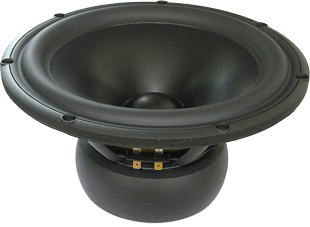open a bigger photo

recommended cabinet 1:
closed cabinet with 34 L volume
from 49/31 Hz (-3dB/-8dB)

recommended cabinet 2:
104 L volume bass reflex cabinet
with HP100 reflex tube, 29 cm long.
from 27/21 Hz (-3dB/-8dB).

## ScanSpeak 26W/8861T00

order no. ss-26W-8861T00

EUR 358,95excl. VAT: € 301.64 / \$ 334.82

24 cm bass driver with paper cone.

• power handling (continuous/programme) = 170 W
• frequency range = 18-1000 Hz
• resonance frequency fs = 19 Hz
• impedance R = 8 Ohm
• sound pressure level SPL = 88,5 dB (2,83V; 1m)
• DC resistance Re = 6,2 Ohm
• force factor BL = 9,9 N/A
• voice coil inductance L = 0,35 mH
• effective piston radiating area Sd = 320 cm2
• effective mechanical mass incl. air load mms = 43,5 g
• equivalent volume of compliance Vas = 231 l
• total Q factor Qts = 0,31 (Qms=5,2, Qes=0,33)
• voice coil diameter = 50 mm
• maximum peak linear excursion vibration xlin = +/- 9 mm
• mounting diameter d = 228 mm
• overall diameter d = 260 mm
• mounting depth (not countersunk) t = 131,7 mm
PDF data sheet

## Classic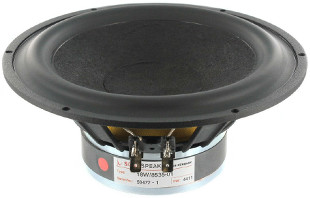open a bigger photo

recommended cabinet 1:
closed cabinet with 17 L volume
from 53/33 Hz (-3dB/-8dB)

recommended cabinet 2:
60 L volume bass reflex cabinet
with HP70 reflex tube, 19 cm long.
from 27/21 Hz (-3dB/-8dB).

## ScanSpeak 18W/8535-01

order no. ss-18W-8535-01

EUR 180,42excl. VAT: € 151.61 / \$ 168.29

17 cm bass-midrange driver with paper cone.

• power handling (continuous/programme) = 70/190 W
• frequency range = 30-3000 Hz
• resonance frequency fs = 25 Hz
• impedance R = 8 Ohm
• sound pressure level SPL = 87,2 dB (2,83V; 1m)
• DC resistance Re = 5,85 Ohm
• force factor BL = 5,9 N/A
• voice coil inductance L = 0,33 mH
• effective piston radiating area Sd = 145 cm2
• effective mechanical mass incl. air load mms = 17,2 g
• equivalent volume of compliance Vas = 68,9 l
• total Q factor Qts = 0,38 (Qms=2,1, Qes=0,46)
• voice coil diameter = 38 mm
• maximum peak linear excursion vibration xlin = +/- 5 mm
• mounting diameter d = 158 mm
• overall diameter d = 177 mm
• mounting depth (not countersunk) t = 74,5 mm
PDF data sheetopen a bigger photo

recommended cabinet 1:
closed cabinet with 3,4 L volume
from 107/68 Hz (-3dB/-8dB)

recommended cabinet 2:
7,2 L volume bass reflex cabinet
with HP50 reflex tube, 23 cm long.
from 68/50 Hz (-3dB/-8dB).

## ScanSpeak 18W/8542-00

order no. ss-18W-8542-00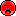- not available

17 cm bass-midrange driver with paper cone.

• power handling (continuous/programme) = 70 W
• frequency range = 30-3000 Hz
• resonance frequency fs = 30 Hz
• impedance R = 8 Ohm
• sound pressure level SPL = 89 dB (2,83V; 1m)
• DC resistance Re = 5,5 Ohm
• force factor BL = 7,8 N/A
• voice coil inductance L = 0,2 mH
• effective piston radiating area Sd = 135 cm2
• effective mechanical mass incl. air load mms = 15 g
• equivalent volume of compliance Vas = 47,9 l
• total Q factor Qts = 0,23 (Qms=1,7, Qes=0,26)
• voice coil diameter = 42 mm
• maximum peak linear excursion vibration xlin = +/- 6,5 mm
• mounting diameter d = 158 mm
• overall diameter d = 177 mm
• mounting depth (not countersunk) t = 77,5 mm
PDF data sheetopen a bigger photo

recommended cabinet 1:
closed cabinet with 5,5 L volume
from 80/50 Hz (-3dB/-8dB)

recommended cabinet 2:
15 L volume bass reflex cabinet
with HP50 reflex tube, 19 cm long.
from 45/34 Hz (-3dB/-8dB).

## ScanSpeak 18W/8545K00

order no. ss-18W-8545K00

EUR 144,00excl. VAT: € 121.01 / \$ 134.32

17 cm bass-midrange driver with paper cone.

• power handling (continuous/programme) = 100 W
• frequency range = 28-3000 Hz
• resonance frequency fs = 28 Hz
• impedance R = 8 Ohm
• sound pressure level SPL = 87,5 dB (2,83V; 1m)
• DC resistance Re = 5,5 Ohm
• force factor BL = 8,2 N/A
• voice coil inductance L = 0,4 mH
• effective piston radiating area Sd = 145 cm2
• effective mechanical mass incl. air load mms = 20,5 g
• equivalent volume of compliance Vas = 46,4 l
• total Q factor Qts = 0,28 (Qms=5,2, Qes=0,3)
• voice coil diameter = 42 mm
• maximum peak linear excursion vibration xlin = +/- 6,5 mm
• mounting diameter d = 158 mm
• overall diameter d = 177 mm
• mounting depth (not countersunk) t = 78 mm
PDF data sheetopen a bigger photo

recommended cabinet 1:
closed cabinet with 3,6 L volume
from 103/65 Hz (-3dB/-8dB)

recommended cabinet 2:
6,5 L volume bass reflex cabinet
with HP50 reflex tube, 29 cm long.
from 69/51 Hz (-3dB/-8dB).

## ScanSpeak 18W/8545-01

order no. ss-18W-8545-01

EUR 178,86excl. VAT: € 150.30 / \$ 166.84

17 cm bass-midrange driver with paper cone.

• power handling (continuous/programme) = 100 W
• frequency range = 25-3000 Hz
• resonance frequency fs = 25 Hz
• impedance R = 8 Ohm
• sound pressure level SPL = 88 dB (2,83V; 1m)
• DC resistance Re = 6,2 Ohm
• force factor BL = 8,4 N/A
• voice coil inductance L = 0,39 mH
• effective piston radiating area Sd = 145 cm2
• effective mechanical mass incl. air load mms = 18 g
• equivalent volume of compliance Vas = 68,6 l
• total Q factor Qts = 0,19 (Qms=1,55, Qes=0,22)
• voice coil diameter = 42 mm
• maximum peak linear excursion vibration xlin = +/- 6,5 mm
• mounting diameter d = 158 mm
• overall diameter d = 177 mm
• mounting depth (not countersunk) t = 77,5 mm
PDF data sheetopen a bigger photo

recommended cabinet 1:
closed cabinet with 20 L volume
from 52/33 Hz (-3dB/-8dB)

recommended cabinet 2:
60 L volume bass reflex cabinet
with HP70 reflex tube, 21 cm long.
from 28/22 Hz (-3dB/-8dB).

## ScanSpeak 21W/8555-00

order no. ss-21W-8555-00

EUR 195,36excl. VAT: € 164.17 / \$ 182.23

21 cm bass-midrange driver with paper cone.

• power handling (continuous/programme) = 100 W
• frequency range = 20-1500 Hz
• resonance frequency fs = 20 Hz
• impedance R = 8 Ohm
• sound pressure level SPL = 87 dB (2,83V; 1m)
• DC resistance Re = 5,5 Ohm
• force factor BL = 8,2 N/A
• voice coil inductance L = 0,4 mH
• effective piston radiating area Sd = 220 cm2
• effective mechanical mass incl. air load mms = 32 g
• equivalent volume of compliance Vas = 134 l
• total Q factor Qts = 0,31 (Qms=4,5, Qes=0,33)
• voice coil diameter = 42 mm
• maximum peak linear excursion vibration xlin = +/- 6,5 mm
• mounting diameter d = 194 mm
• overall diameter d = 222 mm
• mounting depth (not countersunk) t = 89 mm
PDF data sheet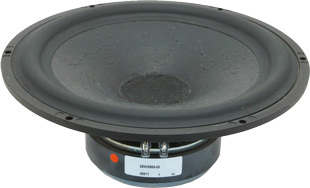open a bigger photo

recommended cabinet 1:
closed cabinet with 66 L volume
from 39/25 Hz (-3dB/-8dB)

recommended cabinet 2:
250 L volume bass reflex cabinet
with HP100 reflex tube, 16 cm long.
from 19/16 Hz (-3dB/-8dB).

## ScanSpeak 25W/8565-00

order no. ss-25W-8565-00

EUR 258,00excl. VAT: € 216.81 / \$ 240.66

24 cm bass driver with paper cone.

• power handling (continuous/programme) = 100 W
• frequency range = 20-600 Hz
• resonance frequency fs = 20 Hz
• impedance R = 8 Ohm
• sound pressure level SPL = 88 dB (2,83V; 1m)
• DC resistance Re = 5,5 Ohm
• force factor BL = 8,2 N/A
• voice coil inductance L = 0,4 mH
• effective piston radiating area Sd = 330 cm2
• effective mechanical mass incl. air load mms = 43 g
• equivalent volume of compliance Vas = 225 l
• total Q factor Qts = 0,41 (Qms=5,4, Qes=0,44)
• voice coil diameter = 42 mm
• maximum peak linear excursion vibration xlin = +/- 6,5 mm
• mounting diameter d = 227 mm
• overall diameter d = 255 mm
• mounting depth (not countersunk) t = 94,5 mm
PDF data sheet

## Discovery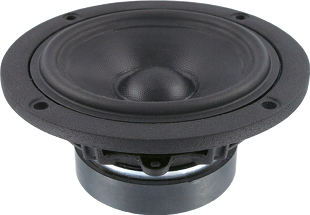open a bigger photo

recommended cabinet 1:
closed cabinet with 0,97 L volume
from 142/90 Hz (-3dB/-8dB)

recommended cabinet 2:
2,7 L volume bass reflex cabinet
with HP35 reflex tube, 17 cm long.
from 81/61 Hz (-3dB/-8dB).

## ScanSpeak 12W/4524G00

order no. ss-12W-4524G00

EUR 49,00excl. VAT: € 41.18 / \$ 45.71

11 cm bass-midrange driver with glass fibre cone.

• power handling (continuous/programme) = 40/70 W
• frequency range = 50-3000 Hz
• resonance frequency fs = 50 Hz
• impedance R = 4 Ohm
• sound pressure level SPL = 88,8 dB (2,83V; 1m)
• DC resistance Re = 3,1 Ohm
• force factor BL = 4,4 N/A
• voice coil inductance L = 0,4 mH
• effective piston radiating area Sd = 59 cm2
• effective mechanical mass incl. air load mms = 6,1 g
• equivalent volume of compliance Vas = 8,2 l
• total Q factor Qts = 0,28 (Qms=3,35, Qes=0,3)
• voice coil diameter = 25 mm
• maximum peak linear excursion vibration xlin = +/- 3 mm
• mounting diameter d = 100 mm
• overall diameter d = 124,7 mm
• mounting depth (not countersunk) t = 58,4 mm
PDF data sheetopen a bigger photo

recommended cabinet 1:
closed cabinet with 1,3 L volume
from 130/82 Hz (-3dB/-8dB)

recommended cabinet 2:
4,1 L volume bass reflex cabinet
with HP35 reflex tube, 12 cm long.
from 70/54 Hz (-3dB/-8dB).

## ScanSpeak 12W/8524G00

order no. ss-12W-8524G00

EUR 49,00excl. VAT: € 41.18 / \$ 45.71

11 cm bass-midrange driver with glass fibre cone.

• power handling (continuous/programme) = 40/70 W
• frequency range = 60-3000 Hz
• resonance frequency fs = 52 Hz
• impedance R = 8 Ohm
• sound pressure level SPL = 85,8 dB (2,83V; 1m)
• DC resistance Re = 6,6 Ohm
• force factor BL = 5,4 N/A
• voice coil inductance L = 0,6 mH
• effective piston radiating area Sd = 59 cm2
• effective mechanical mass incl. air load mms = 5,6 g
• equivalent volume of compliance Vas = 8,2 l
• total Q factor Qts = 0,32 (Qms=3,42, Qes=0,35)
• voice coil diameter = 25 mm
• maximum peak linear excursion vibration xlin = +/- 3 mm
• mounting diameter d = 100 mm
• overall diameter d = 124,7 mm
• mounting depth (not countersunk) t = 58,4 mm
PDF data sheet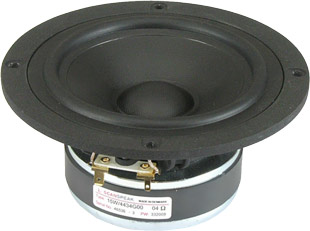open a bigger photo

recommended cabinet 1:
closed cabinet with 1,2 L volume
from 143/90 Hz (-3dB/-8dB)

recommended cabinet 2:
2,9 L volume bass reflex cabinet
with HP35 reflex tube, 15 cm long.
from 85/64 Hz (-3dB/-8dB).

## ScanSpeak 15W/8434G00

order no. ss-15W-8434G00

EUR 65,00excl. VAT: € 54.62 / \$ 60.63

13 cm bass-midrange driver with glass fibre cone.

• power handling (continuous/programme) = 60/120 W
• frequency range = 40-2500 Hz
• resonance frequency fs = 45 Hz
• impedance R = 8 Ohm
• sound pressure level SPL = 86,9 dB (2,83V; 1m)
• DC resistance Re = 5,7 Ohm
• force factor BL = 7,3 N/A
• voice coil inductance L = 0,8 mH
• effective piston radiating area Sd = 80 cm2
• effective mechanical mass incl. air load mms = 8,61 g
• equivalent volume of compliance Vas = 12,8 l
• total Q factor Qts = 0,25 (Qms=3,74, Qes=0,27)
• voice coil diameter = 25 mm
• maximum peak linear excursion vibration xlin = +/- 4,2 mm
• mounting diameter d = 114 mm
• overall diameter d = 149 mm
• mounting depth (not countersunk) t = 69,2 mm
PDF data sheetopen a bigger photo

recommended cabinet 1:
closed cabinet with 0,93 L volume
from 165/104 Hz (-3dB/-8dB)

recommended cabinet 2:
1,9 L volume bass reflex cabinet
with HP35 reflex tube, 18 cm long.
from 106/79 Hz (-3dB/-8dB).

## ScanSpeak 15W/4424G00

order no. ss-15W-4424G00

EUR 49,00excl. VAT: € 41.18 / \$ 45.71

13 cm bass-midrange driver with glass fibre cone.

• power handling (continuous/programme) = 60/120 W
• frequency range = 40-4000 Hz
• resonance frequency fs = 44 Hz
• impedance R = 4 Ohm
• sound pressure level SPL = 90,6 dB (2,83V; 1m)
• DC resistance Re = 2,9 Ohm
• force factor BL = 5,4 N/A
• voice coil inductance L = 0,4 mH
• effective piston radiating area Sd = 80 cm2
• effective mechanical mass incl. air load mms = 8,1 g
• equivalent volume of compliance Vas = 14,5 l
• total Q factor Qts = 0,21 (Qms=3,73, Qes=0,22)
• voice coil diameter = 25 mm
• maximum peak linear excursion vibration xlin = +/- 2,8 mm
• mounting diameter d = 114 mm
• overall diameter d = 149 mm
• mounting depth (not countersunk) t = 68,2 mm
PDF data sheet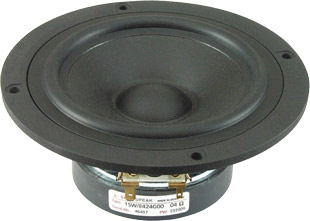open a bigger photo

recommended cabinet 1:
closed cabinet with 1,3 L volume
from 146/92 Hz (-3dB/-8dB)

recommended cabinet 2:
3,3 L volume bass reflex cabinet
with HP35 reflex tube, 13 cm long.
from 87/65 Hz (-3dB/-8dB).

## ScanSpeak 15W/8424G00

order no. ss-15W-8424G00

EUR 49,00excl. VAT: € 41.18 / \$ 45.71

13 cm bass-midrange driver with glass fibre cone.

• power handling (continuous/programme) = 60/120 W
• frequency range = 50-3000 Hz
• resonance frequency fs = 46 Hz
• impedance R = 8 Ohm
• sound pressure level SPL = 87,7 dB (2,83V; 1m)
• DC resistance Re = 5,5 Ohm
• force factor BL = 6,6 N/A
• voice coil inductance L = 0,5 mH
• effective piston radiating area Sd = 80 cm2
• effective mechanical mass incl. air load mms = 7,54 g
• equivalent volume of compliance Vas = 14,5 l
• total Q factor Qts = 0,25 (Qms=3,6, Qes=0,27)
• voice coil diameter = 25 mm
• maximum peak linear excursion vibration xlin = +/- 2,6 mm
• mounting diameter d = 114 mm
• overall diameter d = 149 mm
• mounting depth (not countersunk) t = 68,2 mm
PDF data sheetopen a bigger photo

recommended cabinet 1:
closed cabinet with 0,82 L volume
from 161/102 Hz (-3dB/-8dB)

recommended cabinet 2:
1,7 L volume bass reflex cabinet
with HP35 reflex tube, 22 cm long.
from 104/77 Hz (-3dB/-8dB).

## ScanSpeak 15W/4434G00

order no. ss-15W-4434G00

EUR 57,00excl. VAT: € 47.90 / \$ 53.17

13 cm bass-midrange driver with glass fibre cone.

• power handling (continuous/programme) = 60/120 W
• frequency range = 45-4000 Hz
• resonance frequency fs = 43 Hz
• impedance R = 4 Ohm
• sound pressure level SPL = 89,7 dB (2,83V; 1m)
• DC resistance Re = 3 Ohm
• force factor BL = 5,9 N/A
• voice coil inductance L = 0,5 mH
• effective piston radiating area Sd = 80 cm2
• effective mechanical mass incl. air load mms = 9,6 g
• equivalent volume of compliance Vas = 12,8 l
• total Q factor Qts = 0,21 (Qms=3,69, Qes=0,22)
• voice coil diameter = 32 mm
• maximum peak linear excursion vibration xlin = +/- 4,3 mm
• mounting diameter d = 114 mm
• overall diameter d = 149 mm
• mounting depth (not countersunk) t = 69,2 mm
PDF data sheetopen a bigger photo

recommended cabinet 1:
closed cabinet with 6,9 L volume
from 92/58 Hz (-3dB/-8dB)

recommended cabinet 2:
27 L volume bass reflex cabinet
with HP70 reflex tube, 13 cm long.
from 44/36 Hz (-3dB/-8dB).

## ScanSpeak 18W/8434G00

order no. ss-18W-8434G00

EUR 58,90excl. VAT: € 49.50 / \$ 54.94

16 cm bass-midrange driver with glass fibre cone.

• power handling (continuous/programme) = 55/170 W
• frequency range = 50-2000 Hz
• resonance frequency fs = 50 Hz
• impedance R = 8 Ohm
• sound pressure level SPL = 88,7 dB (2,83V; 1m)
• DC resistance Re = 5,6 Ohm
• force factor BL = 7,2 N/A
• voice coil inductance L = 0,55 mH
• effective piston radiating area Sd = 137 cm2
• effective mechanical mass incl. air load mms = 13,7 g
• equivalent volume of compliance Vas = 19,5 l
• total Q factor Qts = 0,43 (Qms=7,58, Qes=0,46)
• voice coil diameter = 32 mm
• maximum peak linear excursion vibration xlin = +/- 4,2 mm
• mounting diameter d = 144,3 mm
• overall diameter d = 179,6 mm
• mounting depth (not countersunk) t = 81,1 mm
PDF data sheet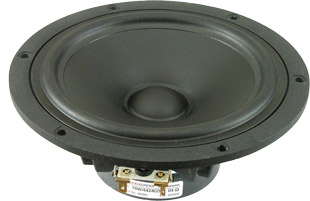open a bigger photo

recommended cabinet 1:
closed cabinet with 6,6 L volume
from 98/62 Hz (-3dB/-8dB)

recommended cabinet 2:
25 L volume bass reflex cabinet
with HP70 reflex tube, 12 cm long.
from 49/39 Hz (-3dB/-8dB).

## ScanSpeak 18W/4424G00

order no. ss-18W4424G00

EUR 52,00excl. VAT: € 43.70 / \$ 48.50

16 cm bass-midrange driver with glass fibre cone.

• power handling (continuous/programme) = 50/110 W
• frequency range = 50-2500 Hz
• resonance frequency fs = 49 Hz
• impedance R = 4 Ohm
• sound pressure level SPL = 90,9 dB (2,83V; 1m)
• DC resistance Re = 3,2 Ohm
• force factor BL = 5,2 N/A
• voice coil inductance L = 0,47 mH
• effective piston radiating area Sd = 137 cm2
• effective mechanical mass incl. air load mms = 11,4 g
• equivalent volume of compliance Vas = 24,1 l
• total Q factor Qts = 0,38 (Qms=4,57, Qes=0,42)
• voice coil diameter = 25 mm
• maximum peak linear excursion vibration xlin = +/- 2,8 mm
• mounting diameter d = 144,3 mm
• overall diameter d = 179,6 mm
• mounting depth (not countersunk) t = 80,1 mm
PDF data sheetopen a bigger photo

recommended cabinet 1:
closed cabinet with 8,2 L volume
from 95/60 Hz (-3dB/-8dB)

recommended cabinet 2:
33 L volume bass reflex cabinet
with HP70 reflex tube, 9 cm long.
from 45/37 Hz (-3dB/-8dB).

## ScanSpeak 18W/8424G00

order no. ss-18W-8424G00

EUR 52,00excl. VAT: € 43.70 / \$ 48.50

16 cm bass-midrange driver with glass fibre cone.

• power handling (continuous/programme) = 50/110 W
• frequency range = 50-2500 Hz
• resonance frequency fs = 54 Hz
• impedance R = 8 Ohm
• sound pressure level SPL = 89,4 dB (2,83V; 1m)
• DC resistance Re = 5,7 Ohm
• force factor BL = 6,6 N/A
• voice coil inductance L = 0,69 mH
• effective piston radiating area Sd = 137 cm2
• effective mechanical mass incl. air load mms = 11,3 g
• equivalent volume of compliance Vas = 20,3 l
• total Q factor Qts = 0,45 (Qms=4,04, Qes=0,51)
• voice coil diameter = 25 mm
• maximum peak linear excursion vibration xlin = +/- 2,6 mm
• mounting diameter d = 144,3 mm
• overall diameter d = 179,6 mm
• mounting depth (not countersunk) t = 80,1 mm
PDF data sheet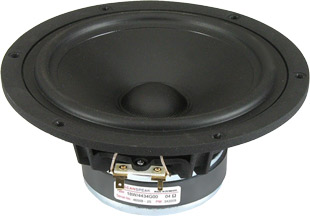open a bigger photo

recommended cabinet 1:
closed cabinet with 4,7 L volume
from 102/64 Hz (-3dB/-8dB)

recommended cabinet 2:
17 L volume bass reflex cabinet
with HP70 reflex tube, 19 cm long.
from 52/41 Hz (-3dB/-8dB).

## ScanSpeak 18W/4434G00

order no. ss-18W-4434G00

EUR 59,80excl. VAT: € 50.25 / \$ 55.78

16 cm bass-midrange driver with glass fibre cone.

• power handling (continuous/programme) = 55/170 W
• frequency range = 50-2000 Hz
• resonance frequency fs = 47 Hz
• impedance R = 4 Ohm
• sound pressure level SPL = 91,3 dB (2,83V; 1m)
• DC resistance Re = 3 Ohm
• force factor BL = 6 N/A
• voice coil inductance L = 0,39 mH
• effective piston radiating area Sd = 137 cm2
• effective mechanical mass incl. air load mms = 14,41 g
• equivalent volume of compliance Vas = 20,7 l
• total Q factor Qts = 0,35 (Qms=7,81, Qes=0,37)
• voice coil diameter = 32 mm
• maximum peak linear excursion vibration xlin = +/- 4,3 mm
• mounting diameter d = 144,3 mm
• overall diameter d = 179,6 mm
• mounting depth (not countersunk) t = 81,1 mm
PDF data sheetopen a bigger photo

recommended cabinet 1:
closed cabinet with 19 L volume
from 69/43 Hz (-3dB/-8dB)

recommended cabinet 2:
63 L volume bass reflex cabinet
with HP100 reflex tube, 23 cm long.
from 36/28 Hz (-3dB/-8dB).

## ScanSpeak 22W/4534G00

order no. ss-22W-4534G00

EUR 82,00excl. VAT: € 68.91 / \$ 76.49

21 cm bass driver with glass fibre cone.

• power handling (continuous/programme) = 70/120 W
• frequency range = 30-2000 Hz
• resonance frequency fs = 30 Hz
• impedance R = 4 Ohm
• sound pressure level SPL = 92,4 dB (2,83V; 1m)
• DC resistance Re = 3 Ohm
• force factor BL = 6 N/A
• voice coil inductance L = 0,33 mH
• effective piston radiating area Sd = 235 cm2
• effective mechanical mass incl. air load mms = 23,7 g
• equivalent volume of compliance Vas = 95 l
• total Q factor Qts = 0,34 (Qms=3,7, Qes=0,37)
• voice coil diameter = 38 mm
• maximum peak linear excursion vibration xlin = +/- 5,7 mm
• mounting diameter d = 185 mm
• overall diameter d = 225 mm
• mounting depth (not countersunk) t = 94 mm
PDF data sheetopen a bigger photo

recommended cabinet 1:
closed cabinet with 24 L volume
from 62/39 Hz (-3dB/-8dB)

recommended cabinet 2:
89 L volume bass reflex cabinet
with HP100 reflex tube, 19 cm long.
from 31/25 Hz (-3dB/-8dB).

## ScanSpeak 22W/8534G00

order no. ss-22W-8534G00

EUR 82,00excl. VAT: € 68.91 / \$ 76.49

21 cm bass driver with glass fibre cone.

• power handling (continuous/programme) = 70/120 W
• frequency range = 30-2000 Hz
• resonance frequency fs = 30 Hz
• impedance R = 8 Ohm
• sound pressure level SPL = 88,8 dB (2,83V; 1m)
• DC resistance Re = 5,9 Ohm
• force factor BL = 7,8 N/A
• voice coil inductance L = 0,56 mH
• effective piston radiating area Sd = 235 cm2
• effective mechanical mass incl. air load mms = 23,1 g
• equivalent volume of compliance Vas = 94,2 l
• total Q factor Qts = 0,39 (Qms=4,14, Qes=0,43)
• voice coil diameter = 38 mm
• maximum peak linear excursion vibration xlin = +/- 5,8 mm
• mounting diameter d = 185 mm
• overall diameter d = 225 mm
• mounting depth (not countersunk) t = 94 mm
PDF data sheetopen a bigger photo

recommended cabinet 1:
closed cabinet with 44 L volume
from 45/28 Hz (-3dB/-8dB)

recommended cabinet 2:
167 L volume bass reflex cabinet
with HP100 reflex tube, 19 cm long.
from 22/18 Hz (-3dB/-8dB).

## ScanSpeak 26W/8534G00

order no. ss-26w-8534-00

EUR 96,00excl. VAT: € 80.67 / \$ 89.55

25 cm bass driver with aluminium cone.

• power handling (continuous/programme) = 90/175 W
• frequency range = 20-1000 Hz
• resonance frequency fs = 23 Hz
• impedance R = 8 Ohm
• sound pressure level SPL = 89 dB (2,83V; 1m)
• DC resistance Re = 5,7 Ohm
• force factor BL = 10,1 N/A
• voice coil inductance L = 0,96 mH
• effective piston radiating area Sd = 350 cm2
• effective mechanical mass incl. air load mms = 50,5 g
• equivalent volume of compliance Vas = 150 l
• total Q factor Qts = 0,4 (Qms=9,12, Qes=0,42)
• voice coil diameter = 38 mm
• maximum peak linear excursion vibration xlin = +/- 6 mm
• mounting diameter d = 231 mm
• overall diameter d = 272 mm
• mounting depth (not countersunk) t = 106 mm
PDF data sheetopen a bigger photo

recommended cabinet 1:
closed cabinet with 35 L volume
from 50/31 Hz (-3dB/-8dB)

recommended cabinet 2:
124 L volume bass reflex cabinet
with HP100 reflex tube, 22 cm long.
from 25/20 Hz (-3dB/-8dB).

## ScanSpeak 26W/4534G00

order no. ss-26W-4534G00

EUR 96,00excl. VAT: € 80.67 / \$ 89.55

25 cm bass driver with aluminium cone.

• power handling (continuous/programme) = 90/175 W
• frequency range = 20-1000 Hz
• resonance frequency fs = 23 Hz
• impedance R = 4 Ohm
• sound pressure level SPL = 90,5 dB (2,83V; 1m)
• DC resistance Re = 3,8 Ohm
• force factor BL = 8,8 N/A
• voice coil inductance L = 0,7 mH
• effective piston radiating area Sd = 350 cm2
• effective mechanical mass incl. air load mms = 52 g
• equivalent volume of compliance Vas = 156 l
• total Q factor Qts = 0,36 (Qms=9,57, Qes=0,37)
• voice coil diameter = 38 mm
• maximum peak linear excursion vibration xlin = +/- 6 mm
• mounting diameter d = 231 mm
• overall diameter d = 272 mm
• mounting depth (not countersunk) t = 106 mm
PDF data sheet

 ASE Tweeter Full range Mids Woofer Subwoofer Car-HiFi no longer available
 Illuminator Revelator Classic Discovery
The cabinet volume calculations are executed considering an external resistance of 0.3 Ohm. We are using, if no other data is known or available, the information supplied by the manufacturer.

Please note: the frequency response doesn't say much about the cut-off frequency inside the cabinet! Therefore, we mostly give you 2 examples with dimensioning considerations and an indication, how low the loudspeaker will reproduce.

Home